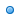# 1 to 12 Multiplication Times Tables Chart##Related Math Calculators

Online math number calculation, formulas , Online Algebra calculation, formulas , Matrix calculation, formulas , Digital calculation, Statistical calculation

##Multiplication Times Tables Chart 1 to 12

An online math times table

1 Times Table 2 Times Table 3 Times Table 4 Times Table
1 x 1 = 1 1 x 2 = 2 1 x 3 = 3 1 x 4 = 4
2 x 1 = 2 2 x 2 = 4 2 x 3 = 6 2 x 4 = 8
3 x 1 = 3 3 x 2 = 6 3 x 3 = 9 3 x 4 = 12
4 x 1 = 4 4 x 2 = 8 4 x 3 = 12 4 x 4 = 16
5 x 1 = 5 5 x 2 = 10 5 x 3 = 15 5 x 4 = 20
6 x 1 = 6 6 x 2 = 12 6 x 3 = 18 6 x 4 = 24
7 x 1 = 7 7 x 2 = 14 7 x 3 = 21 7 x 4 = 28
8 x 1 = 8 8 x 2 = 16 8 x 3 = 24 8 x 4 = 32
9 x 1 = 9 9 x 2 = 18 9 x 3 = 27 9 x 4 = 36
10 x 1 = 10 10 x 2 = 20 10 x 3 = 30 10 x 4 = 40
11 x 1 = 11 11 x 2 = 22 11 x 3 = 33 11 x 4 = 44
12 x 1 = 12 12 x 2 = 24 12 x 3 = 36 12 x 4 = 48
5 Times Table 6 Times Table 7 Times Table 8 Times Table
1 x 5 = 5 1 x 6 = 6 1 x 7 = 7 1 x 8 = 8
2 x 5 = 10 2 x 6 = 12 2 x 7 = 14 2 x 8 = 16
3 x 5 = 15 3 x 6 = 18 3 x 7 = 21 3 x 8 = 24
4 x 5 = 20 4 x 6 = 24 4 x 7 = 28 4 x 8 = 32
5 x 5 = 25 5 x 6 = 30 5 x 7 = 35 5 x 8 = 40
6 x 5 = 30 6 x 6 = 36 6 x 7 = 42 6 x 8 = 48
7 x 5 = 35 7 x 6 = 42 7 x 7 = 49 7 x 8 = 56
8 x 5 = 40 8 x 6 = 48 8 x 7 = 56 8 x 8 = 64
9 x 5 = 45 9 x 6 = 54 9 x 7 = 63 9 x 8 = 72
10 x 5 = 50 10 x 6 = 60 10 x 7 = 70 10 x 8 = 80
11 x 5 = 55 11 x 6 = 66 11 x 7 = 77 11 x 8 = 88
12 x 5 = 60 12 x 6 = 72 12 x 7 = 84 12 x 8 = 96
9 Times Table 10 Times Table 11 Times Table 12 Times Table
1 x 9 = 9 1 x 10 = 10 1 x 11 = 11 1 x 12 = 12
2 x 9 = 18 2 x 10 = 20 2 x 11 = 22 2 x 12 = 24
3 x 9 = 27 3 x 10 = 30 3 x 11 = 33 3 x 12 = 36
4 x 9 = 36 4 x 10 = 40 4 x 11 = 44 4 x 12 = 48
5 x 9 = 45 5 x 10 = 50 5 x 11 = 55 5 x 12 = 60
6 x 9 = 54 6 x 10 = 60 6 x 11 = 66 6 x 12 = 72
7 x 9 = 63 7 x 10 = 70 7 x 11 = 77 7 x 12 = 84
8 x 9 = 72 8 x 10 = 80 8 x 11 = 88 8 x 12 = 96
9 x 9 = 81 9 x 10 = 90 9 x 11 = 99 9 x 12 = 108
10 x 9 = 90 10 x 10 = 100 10 x 11 = 110 10 x 12 = 120
11 x 9 = 99 11 x 10 = 110 11 x 11 = 121 11 x 12 = 132
12 x 9 = 108 12 x 10 = 120 12 x 11 = 132 12 x 12 = 144##Generate Times Table##Recommended Pages##Best Times Tables Generator Here

Our times table creator provides a fun and engaging way for students to learn their times tables.Unlimited Times Table GeneratorCustomize Times Table GeneratorOne click Times Table Answer GeneratorInteractive Times Table Quiz GeneratorOne Hundred Chart